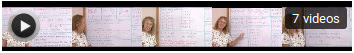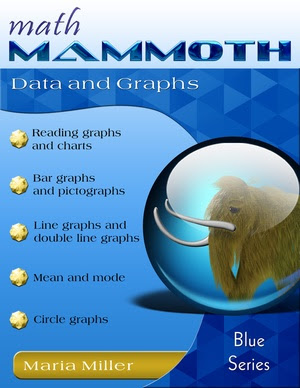## Posts

Showing posts from September, 2015Check out my videos on this page at my site ! They present an organized and logical method for children to learn their addition facts within 10 using PATTERNS that arise from the number system itself. Children LOVE patterns, and patterns are the essence of math — mathematics has even been defined as the "science of patterns". In this method, we study sums with 5, sums with 6, sums with 7 , and so on until sums with 10 (also cal led "number bonds"). These sums or number bonds are not studied in random order, but grouped by the SUM, or the "answer".

### Awesome math dictionary/glossaryHere's an awesome math dictionary or glossary that your children will LOVE browsing :^) Math Dictionary for Kids (original, for computers only)  Or Math Dictionary - Quick Reference (also for tablets) Enjoy!

### Lessons on integer basics (pre-algebra/7th grade math)Here's a collection of 7 video lessons of mine on the basics of integer arithmetic, meant for pre-algebra or 7th grade math: Click the image to access a Youtube playlist for those 7 videos. It contains the following individual video lessons, which you can also access individually (on my site): Basics of integers Review of adding integers : counters, number line, different signs, rules How to turn a subtraction of integers into addition — and WHY it works The rules for multiplying integers — and WHY they work (incl. negative times negative and exponents) Division of Integers - basic lesson for pre-algebra/7th grade math Integers lesson: solved examples, part 1 and part 2 (2 videos)

### New book in the Blue Series: Data and GraphsA new kid on the block! Math Mammoth Data and Graphs is a worktext that covers common statistical graphs and some related topics for grades 2-5. It is a worktext, which means it includes both the "text" (instruction) and the "work" (exercises and problems). The book starts with the easiest topics: reading and making bar graphs, pictograms, histograms, and various kinds of line graphs .  Toward the end of the book, we also study average (also called the mean) and mode , and how these two concepts relate to line and bar graphs. Sample pages (PDF) Contents and Introduction Making Bar Graphs, part 2 Reading Line Graphs Mean, Mode, and Bar Graphs Average Prices & ordering PDF download \$4.20 Buy at Kagi